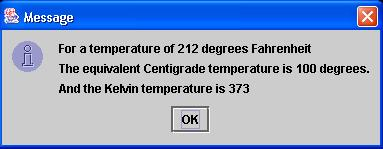### Converting Temperatures

6 points

Introduction
In this program, you ask the user for a Fahrenheit temperature and then you output that temperature, converted to Centrigrade and Kelvin. A program run might look like this:
What is the Fahrenheit temperature? 100

For a temperature of 100 degrees Fahrenheit:
The equivalent Centigrade temperature is 37.78 degrees.
And the Kelvin temperature is 310.78.
Press any key to continue...

Other Parts of the Program
Once you download 'convertTemps.java' (see 'Starting Point' below), notice the line 'private double Fahr;'. This line creates a variable 'Fahr' of type 'double'. 'Fahr' stores the Fahrenheit temperature that your program converts to Centigrade and Kelvin.

Also notice these lines:
public convertTemps(double fahr)
{    Fahr = fahr;
}
This is called a 'constructor' and it runs when the object 'tempConverter' is created in 'main()'. It accepts as input the variable 'fahr,' sent from 'main(),' and sets the variable 'Fahr' equal to 'fahr'. So if the user types in 100 as the temperature she wants to convert, first 'fahr' equals 100 and then 'Fahr' is also set to 100.

main(String[] args)
In 'main(String[] args)', you see this line:
input = JOptionPane.showInputDialog("");
This line causes a Dialog box to pop up which the user can use to type in the temperature. Put the question you are asking the user inside the double-quotes.

fahr = Integer.parseInt(input);
This line converts a String variable (input) to an integer variable (fahr).

convertTemps tempConverter = new convertTemps(fahr);
This line creates an object called 'tempConverter' of type 'convertTemps' -- the name of the class.

tempConverter.calculateTemps();
This line calls the 'calculateTemps()' method that you are writing.

System.exit(0);
This line is necessary to close the 'thread' that the earlier call to 'JOptionPane.showInputDialog()' opened.

calculateTemps()
Writing this method is your job. To convert from Fahrenheit to Centigrade, first subtract 32 from the Fahrenheit temperature, then multiply the result by 5/9. To convert from Centigrade to Kelvin, add 273.
In this method you see a String variable 'msg' declared. The next 3 lines are where 'msg' is set up. The syntax would be like this:
msg = msg + "The equivalent Centigrade temperature is " + Cent + " degrees.\n";
Finally, a call to 'JOptionPane.showMessageDialog(null, msg);' displays 'msg'. You should get output that looks like this:Good Fahrenheit temperatures to use to test your program include -40°, 32° and 212° F. At -40° F, the Fahrenheit and Centigrade temperature scales meet.

### Extra Credit

1 point

If you want to get a little fancy and set the number of decimal digits that print to 2, add this code to 'calculateTemps()':
NumberFormat f = NumberFormat.getNumberInstance();
f.setMaximumFractionDigits(2);
Then whenever you print out a 'double,' put that variable inside 'f.format()'. For example, if you have a 'double' called 'mpg,' this line would cause 'mpg' to print with 2 decimal digits:
System.out.println("Miles per gallon: " + f.format(mpg) );
You also have to add the line 'import java.text.NumberFormat;' at the top of the program.